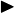In the previous blogs, we have discussed Logistic Regression and its assumptions. Today, the main topic is the theoretical and empirical goods and bads of this model.Simplicity and transparency. Logistic Regression is just a bit more involved than Linear Regression, which is one of the simplest predictive algorithms out there. It is also transparent, meaning we can see through the process and understand what is going on at each step, contrasted to the more complex ones (e.g. SVM, Deep Neural Nets) that are much harder to track.Giving probabilistic output. Some other algorithms (e.g. Decision Tree) only produce the most seemingly matched label for each data sample, meanwhile, Logistic Regression gives a decimal number ranging from 0 to 1, which can be interpreted as the probability of the sample to be in the Positive Class. With that, we know how confident the prediction is, leading to a wider usage and deeper analysis.Feature importance and direction. There are not many models that can provide feature importance assessment, among those, there are even lesser that can give the direction each feature affects the response value – either positively or negatively (e.g. Decision Tree can show feature importances, but not able to tell the direction of their impacts). Fortunately, Logistic Regression is able to do both. Looking at the coefficient weights, the sign represents the direction, while the absolute value shows the magnitude of the influence.Able to do online-learning. Logistic Regression models are trained using the Gradient Accent, which is an iterative method that updates the weights gradually over training examples, thus, supports online-learning. Compared to those who need to be re-trained entirely when new data arrives (like Naive Bayes and Tree-based models), this is certainly a big plus point for Logistic Regression.The assumption of linearity in the logit can rarely hold. It is usually impractical to hope that there are some relationships between the predictors and the logit of the response. However, empirical experiments showed that the model often works pretty well even without this assumption.Uncertainty in Feature importance. This trait is very similar to that of Linear regression. While the weight of each feature somehow represents how and how much the feature interacts with the response, we are not so sure about that. The weight does not only depend on the association between an independent variable and the dependent variable, but also the connection with other independent variables.Not robust to big-influentials. As we have elaborated in the post about Logistic Regression’s assumptions, even with a small number of big-influentials, the model can be damaged sharply. It is essential to pre-process the data carefully before giving it to the Logistic model.Require more data. This is also explained in previous posts: A guideline for the minimum data needed is 10 data points for each predictor variable with the least frequent outcome. While Deep Learning usually requires much more data than Logistic Regression, other models, especially the generative models (like Naive Bayes) need much less.

However, if we can provide enough data, the model will work well. Apart from actually collecting more, we could consider data augmentation as a means of getting more with little cost.

References: